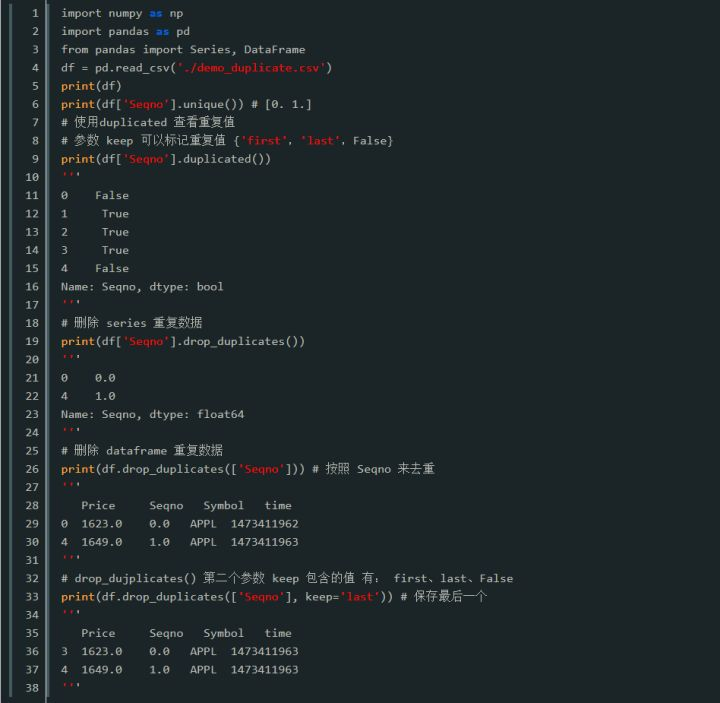• 今天小编就为大家分享一篇python去重,一个由dict组成的list的去重示例，具有很好的参考价值，希望对大家有所帮助。一起跟随小编过来看看吧
• 数据去重可以使用duplicated()和drop_duplicates()两个方法。 DataFrame.duplicated（subset = None，keep =‘first’）返回boolean Series表示重复行 参数： subset：列标签或标签序列，可选 仅考虑用于标识重复项...数据去重可以使用duplicated()和drop_duplicates()两个方法。
DataFrame.duplicated（subset = None，keep =‘first’）返回boolean Series表示重复行
参数：
subset：列标签或标签序列，可选
仅考虑用于标识重复项的某些列，默认情况下使用所有列
keep：{‘first’，‘last’，False}，默认’first’
first：标记重复，True除了第一次出现。
last：标记重复，True除了最后一次出现。
错误：将所有重复项标记为True。
相关推荐：《0基础 Python 入门教程》相关免费资料分享（点击即可免费观看~）
0基础入门 Python
一小时全面认识Python爬虫开发
快速学习python基础
142244252 学习资料交流群，想要和志同道合的朋友一起学习，大家互相分享自己的学习资料和作品，欢迎感兴趣的朋友共同学习，共同进步，每天还会有免费的公开课程！！
展开全文数据 编程语言 程序
• 3) L2 = list(L1) L3 = [] for i in L2: if i not in L3: L3.append(i) print(L3) 结果为： [1, 2, 3] 以下来自博客：Python 二维数组元素去重 np.unique()函数的使用 import numpy as np # 4.2.1 一维数组去重 a =...
 栗子1：利用 set
L1 = (1, 1, 2, 2, 3, 3)
print(set(L1))

结果为： {1, 2, 3}
知识点拓展：集合元素的访问
集合元素可以通过转化为列表来进行访问，对于集合，存在交集、并集、差集等等很多操作。
L1 = {1, 2, 5}
L2 = list(L1)
print(L2)


栗子2：利用 np.unique
import numpy as np
L1 = (1, 1, 2, 2, 3, 3)
print(np.unique(L1))

结果为： [1 2 3]

栗子3：利用循环
import numpy as np
L1 = (1, 1, 2, 2, 3, 3)
L2 = list(L1)
L3 = []

for i in L2:
if i not in L3:
L3.append(i)
print(L3)

结果为： [1, 2, 3]

以下来自博客：Python 二维数组元素去重 np.unique()函数的使用
import numpy as np

# 4.2.1 一维数组去重
a = np.array([1, 2, 3, 4, 5, 5, 7, 3, 2, 2, 8, 8])
print('去重前：', a)  # 去重前： [1 2 3 4 5 5 7 3 2 2 8 8]
b = np.unique(a)
print('去重后：', b)  # 去重后： [1 2 3 4 5 7 8]

# 4.2.2 二维数组去重
c = np.array(((1, 2), (3, 4), (5, 6), (1, 3), (3, 4), (7, 6)))
print('去重前：', c)
# 方法一：利用set,set是无序不重复集合(推荐)
s = set() #创建空集合
for t in c:
g = np.array(list(s)) # 将集合转换为列表，最后转为二维数组
print('去重后：', g)
# 合起来写
print('方法一去重后：', np.array(list(set(tuple(t) for t in c))))
# 方法二：转换为虚数
r, i = np.split(c, (1, ), axis=1)
print(r)
print(i)
x = r + i * 1j
# x = c[:, 0] + c[:, 1] * 1j
print('转换成虚数：', x)  # 变成一维数组，可使用np.unique()
print('虚数去重后：', np.unique(x))
print(np.unique(x, return_index=True, return_counts=True))
# return_index=True表示返回另一个参数，这个参数也是一个数组，
# array([0, 3, 1, 2, 5]表示去重后的每个元素在去重前的数组中的位置/下标
# return_counts=True表示返回各元素出现的频次
idx = np.unique(x, return_index=True)
# 第列的数组正是return_index=True返回的内容：在c中的位置/下标
print('二维数组去重：\n', c[idx]) #提取这些下标所在的元素


字符串去重： python中join函数
展开全文• python去重列表，并获取重复值索引 先上代码 a = [1, 2, 12, 1, 2, 1, 3, 4, 5, 4, 3, 2] b = list(set(a)) print(b) for target in b: for index, nums in enumerate(a): if nums == target: print(target,index...


这里写自定义目录标题
python去重列表，并获取重复值索引
先上代码

a = [1, 2, 12, 1, 2, 1, 3, 4, 5, 4, 3, 2]
b = list(set(a))
print(b)
for target in b:
for index, nums in enumerate(a):
if nums == target:
print(target,index)
在这里插入代码片

列表b是去重后的列表 执行后结果：
[1, 2, 3, 4, 5, 12]
1 0
1 3
1 5
2 1
2 4
2 11
3 6
3 10
4 7
4 9
5 8
12 2

进程已结束,退出代码0


这里参考了大神的写法，加入了去重和赋值

这里是引用

https://blog.csdn.net/weixin_44786231/article/details/89098002 另外我觉得这个讲去重的挺不错的 python 列表去重(数组)的几种方法

这里是引用

https://www.cnblogs.com/zknublx/p/6042295.html
展开全文列表
drop_duplicate(subset,keep,inplace)    #去重函数
subset:子列名
keep:保留第一列或最后一列,first/last
inplace：是否在本数据集修改
示例：
df.drop(subset=['item_id','buyer_admin_id'],keep='first')

#keep参数保证保留的是第一次重复的列还是最后一次重复的列

展开全文• numbers = [1,1,7,7,7,3,2,5,6,2,4,6,2,3] unique = list(set(numbers)) print(unique) print(len(unique)) [1, 2, 3, 4, 5, 6, 7] 7
• 今天小编就为大家分享一篇Python 列表去重去除空字符的例子，具有很好的参考价值，希望对大家有所帮助。一起跟随小编过来看看吧
• python去重、根据某列统计另一列频数 假如有如下格式的数据，实现两个操作：（1）根据“ABC”列去重；（2）根据"jd"列统计“cun” 代码如下： （1）去重 data1=data.drop_duplicates('ABC') # 删除掉重复的行...
• 今天小编就为大家分享一篇对python列表里的字典元素去重方法详解，具有很好的参考价值，希望对大家有所帮助。一起跟随小编过来看看吧
• python可以说2017比较火的一个语言了，下面这篇文章主要给大家介绍了关于利用Python实现嵌套列表去重的相关资料，文中通过示例代码介绍的非常详细，需要的朋友可以参考借鉴，下面来一起看看吧。
• 使用方法，将要去重的部分保存成为csv格式，并命名为all.csv，直接执行后，会在当前目录下生成qc.csv的文件！ #coding:utf-8 ciku=open(r'all.csv','r') #打开需要去重文件 xieci=open(r'qc.csv','w') #打开处理后...
• 由于在python 爬虫过程中有爬取到重复的链接，并没有进行去重操作，故，这里再通过python自带的set再次进行去重一次。 下面是给出来的代码 # -*- coding: utf-8 -*- # @Time : 2018/9/21 13:31 # @Author : 蛇崽 ...json json去重 数据去重
• 下面小编就为大家分享一篇python对excel文档去重及求和的实例，具有很好的参考价值，希望对大家有所帮助。一起跟随小编过来看看吧
• Python字符串操作常用函数，包含了检索、统计、分割、替换、大小写转换、对齐，空格删除、字符串判断（头尾+组成）
•  top = 1 i = 1 def bubble_sort(a): global top for i in range(len(a)-1): ...本人初学python的菜鸟一枚。我想实现去重并升序。我知道有更好的方法，但我想知道按这个思路怎么改。谢谢！
• 主要介绍了python读取TXT到数组及列表去重后按原来顺序排序的方法,涉及Python操作txt文件、列表去重及排序的相关技巧,需要的朋友可以参考下
• 一、使用集合直接去重 ids = [1,4,3,3,4,2,3,4,5,6,1]ids = list(set(ids)) 处理起来比较简单，使用了集合方法set进行处理，不过结果不会保留之前的顺序。 二、列表法 ids = [1,2,3,3,4,2,3,4,5,6,1]news_i...
• https://pypi.org/project/xlrd/ xlrd是1.2的版本 https://pypi.org/project/xlwt/ 分别下载tar.gz 文件 解压后的文件夹，cmd python setup.py install
• 1 List: 1 2 3 listA = ['python','python','言','是','一','门','动','态','语','言'] print sorted(set(listA), key = listA.index) DataFrame： 1 ...
• 数据去重可以使用duplicated()和drop_duplicates()两个方法。DataFrame.duplicated(subset = None，keep =‘first’)返回boolean Series表示重复行参数：subset：列标签或标签序列，可选仅考虑用于标识重复项的某些...
• ## Python数据去重

千次阅读 2019-04-12 09:07:21
普通去重： """ 去重并按原文件顺序排序 """ from time import time ...for line in open(r'e:/Python/mypy/test.txt', 'r+'): new_list.append(line) new_list2 = list(set(new_l...
• @python列表去重计数TOC python import pandas as pd import numpy as np from pprint import pprint list = ['A', 'B', 'B', 'c'] #对标签进行workcount Klist, Vcount = np.unique(list,return_counts=True) ...
• ## python pandas 去重

千次阅读 2018-08-13 15:32:12
newdata.drop_duplicates(subset=['A','B','C','D'],keep=False) 当keep=False时，就是去掉所有的重复行 当keep=‘first’时，就是保留第一次出现的重复行 当keep=’last’时就是保留最后一次出现的重复行。...pandas
• python3数据去重（pandas） 去重操作是我们处理数据的时候经常遇到的！ 接下来告诉大家，仅仅用几行代码就可以实现的去重操作 这边会用到pandas库 这是一个非常非常强大的库，这里面有着处理数据特别简单方便的方法...数据去重 pandas去重 python数据去重 pandas数据去重
• 列表去重 新列表搜集法 创建新列表 遍历原理表的所有元素，若该元素不在新列表中，将元素添加到新列表中 分析：该方式可保证元素顺序 import random src_list = [random.randint(20, 30) for i in range(15)] ...
• l1 = ['b','c','d','b','c','a','a'] l2 = [] [l2.append(i) for i in l1 if not i in l2] print l2...

# python去重python 订阅